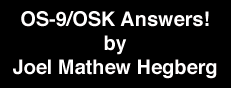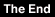##March 1994 issue

Welcome back! In my last issue I left off giving some C source code for playing a digital sound under K-Windows. This time, I'm pleased to write that Ted Jaeger has sent me some BASIC source code ("play.bas" -- listing #1) to demonstrate playing .IFF sound files under K-Windows. Thanks, Ted! Play.bas requires the two subroutines "htoi.bas" and "itoh.bas" (listings #2 & #3, respectively) to help determine the IFF sound file header information.

One advantage to using C over BASIC for a 'play' function is memory allocation. Using C, a programmer can dynamically allocate memory to store a sound in memory (see my column in the last issue for an example). With BASIC, you're pretty much stuck with the amount of memory you received at run-time. Worse, under OSk, the user is the one that has to remember to request additional memory, if needed. Ted handles this BASIC shortfall very nicely by using the windowing system to dynamically allocate the memory needed for the sound to reside in. By using K-Window's GetBlk function (\$1b \$2c) and some strategic SysCall's, "play.bas" defines a get/put buffer, determines its memory address, reads in the digital sound data, starts the sound sample playing , and then releases the buffer memory back to the system.

One interesting note, under MM/1 K-Windows digital sound is controlled by a DMA sound chip which takes care of all the sound output, thus freeing up the system's CPU for other tasks at hand. When Ted's program exits, the sound sample is still probably not finished playing (unless it's extremely short -- as in less than a second in length), yet it's memory is deallocated when the program exits. Will this cause a problem? Not really. The worst thing that can happen under this circumstance would be for another process to allocate the same memory the sound sample is occupying, and write over the sound sample before it's finished playing. In that instance, the sound data would be corrupted, and you would hear garbage for the remaining play duration. In my experience, this is a rare case scenario -- but rest assured you can induce such a occurrence if your heart is set on it!

```Listing #1 -- "play.bas"
========================
PROCEDURE play
(* subroutine to play brief digitized sounds saved
(* in monophonic iff format
(* call play passing complete path name of
(* the sound file e.g., /dd/snds/ding.iff
(* if packed run with new RunB (data size=8000 bytes)
(* December 15, 1993

PARAM filename:STRING

(* register mask for system calls
TYPE registers=d(8),a(8),pc:INTEGER
DIM regs:registers
DIM callcode:INTEGER

(* avoid BGFX by setting up variables
(* to PUT graphics commands
DIM openbuf(12):BYTE
openbuf(1)=\$1b
openbuf(2)=\$2c
DIM kilbuf(4):BYTE
kilbuf(1)=\$1b
kilbuf(2)=\$2a

(* array for first 34 bytes of sound file header
(* we'll find length and frequency info here

(* variables for integer to hex and
(* hex to integer conversions
DIM x,y,z:BYTE
DIM x\$,y\$,z\$:STRING
DIM hex:STRING

(* sound file buffer data
DIM bufsize:INTEGER
DIM buflocate:INTEGER
DIM pixels:INTEGER

(* process id needed so
DIM id:INTEGER
DIM group:BYTE

DIM path:INTEGER

ON ERROR GOTO 1000

(* get the process id
(* for group number of buffer
callcode=\$0c
RUN syscall(callcode,regs)
id=regs.d(1)
group=id

OPEN #path,filename
CLOSE #path

(* get the buffer size of sound file
RUN itoh(x,hex)
x\$=hex
RUN itoh(y,hex)
y\$=hex
RUN itoh(z,hex)
z\$=hex

(* if sound file too large abort
IF bufsize>53248 THEN
END "Sound file too large"
ENDIF

(* get sampling frequency of sound file
RUN itoh(x,hex)
x\$=hex
RUN itoh(y,hex)
y\$=hex

(* open a buffer to hold sound file
(* size of buffer determined in offset
(* 9, 10, 11, 12 of openbuf array
(* x dimension of buffer fixed at 512
(* y dimension of buffer varies to allow proper size
(* for holding sound file
(* size calculated by multiplying # of bytes in buffer X 2
(* this indicates # of needed pixels in buffer
(* because in Type 0 screen there are 2 pixels per byte
pixels=bufsize*2

(* since 512 pixels per row we can divide total pixels
(* by 512 to determine how many rows are needed in the
(* open buffer call
y=pixels/512

(* cant exceed max number of rows on screen
IF y>208 THEN
y=208
ENDIF

openbuf(3)=group
openbuf(4)=1
openbuf(5)=0
openbuf(6)=0
openbuf(7)=0
openbuf(8)=0
openbuf(9)=2
openbuf(10)=0
openbuf(11)=0
openbuf(12)=y
PUT #1,openbuf

(* map the buffer
callcode=\$8d
regs.d(1)=1
regs.d(2)=\$a9
regs.d(3)=group*256+1
RUN syscall(callcode,regs)
buflocate=regs.a(1)

(* open and read in entire sound file
OPEN #path,filename
callcode=\$89
regs.d(1)=path
regs.d(2)=bufsize
regs.a(1)=buflocate
RUN syscall(callcode,regs)
CLOSE #path

(* play sound file
callcode=\$8e
regs.d(1)=1
regs.d(2)=\$97
regs.d(3)=bufsize
regs.d(4)=freq/2
regs.d(5)=0
regs.d(6)=0
regs.d(7)=0
regs.a(1)=buflocate
RUN syscall(callcode,regs)

(* kill the buffer holding the sound file
(* VERY important because sound buffers are
(* large and RUNB limits data memory  to 8000 bytes
kilbuf(3)=group
kilbuf(4)=1
PUT #1,kilbuf

END

errnum=ERR
PRINT "got error "; errnum

Listing #2 -- "htoi.bas"
========================
PROCEDURE htoi
(* convert a 6 digit or smaller
(* hex value to integer
(* hex value received as a string
(* in hex\$
(* December 15, 1993

PARAM hex\$:STRING

DIM digit(6):INTEGER

DIM l\$:STRING
DIM l:INTEGER

DIM count:INTEGER

(* examine digits in hex\$ individually
FOR count=1 TO LEN(hex\$)
l\$=MID\$(hex\$,count,1)

(* and convert them to integer format
IF l\$="a" OR l\$="A" THEN
l=10
ELSE
IF l\$="b" OR l\$="B" THEN
l=11
ELSE
IF l\$="c" OR l\$="C" THEN
l=12
ELSE
IF l\$="d" OR l\$="D" THEN
l=13
ELSE
IF l\$="e" OR l\$="E" THEN
l=14
ELSE
IF l\$="f" OR l\$="F" THEN
l=15
ELSE
l=VAL(l\$)
ENDIF
ENDIF
ENDIF
ENDIF
ENDIF
ENDIF

(* now get the value of each digit
digit(count)=l*16^(LEN(hex\$)-count)

NEXT count

(* now find total of converted hex values
FOR count=1 TO LEN(hex\$)
NEXT count

END

Listing #3 -- "itoh.bas"
========================
PROCEDURE itoh
(* Convert a value < 256 to hex
(* December 15, 1993

PARAM value:BYTE

DIM x:INTEGER
DIM x\$:STRING
DIM digit1,digit2:STRING

(* get digit in 16s place first
x=value/16
GOSUB 10
digit1=x\$

(* now get digit in 1s place
x=MOD(value,16)
GOSUB 10
digit2=x\$

(* now add the two digits to get hex value

END

10 (* get one hex digit of the answer
IF x=15 THEN
x\$="f"
ELSE
IF x=14 THEN
x\$="e"
ELSE
IF x=13 THEN
x\$="d"
ELSE
IF x=12 THEN
x\$="c"
ELSE
IF x=11 THEN
x\$="b"
ELSE
IF x=10 THEN
x\$="a"
ELSE
x\$=STR\$(x)
ENDIF
ENDIF
ENDIF
ENDIF
ENDIF
ENDIF

RETURN
```

Ted has also sent me some source for creating a fancy shell window from within BASIC (listing #4). For those who prefer to program under C, I've ported it for you and included the source (listing #5). The window which is created for the shell is NOT an overlay window, but rather is an independent window, which can be moved, resized, or pushed back. To close up the shell window, just hit the 'Esc' key. Ted stayed away from direct BGFX calls for his BASIC source, so I stayed away from the CGFX library calls in my C version, just in case anyone thought you absolutely _had_ to use CGFX library calls to do K-Windows screen functions.

```Listing #4 -- "popup.bas"
=========================
PROCEDURE popup
(* place a sizeable, relocatable, push-back
(* window on the current Type 0 screen
(* press ESC key to exit pop up window
(* December 16, 1993

(* register mask for system calls
TYPE registers=d(8),a(8):INTEGER
DIM regs:registers

(* variables to make and add features to the new window
(* let's avoid forking shells or calling BGFX
(* so we'll set up to PUT the commands
DIM dwset(9):BYTE
DIM frame(3):BYTE
DIM move(21):BYTE
DIM shape(22):BYTE
DIM push(21):BYTE

(* variables to dup paths
DIM oldpath(3):INTEGER
DIM newpath:INTEGER
DIM path:INTEGER
DIM I_Dup:INTEGER
I_Dup=\$82

DIM errnum:INTEGER

ON ERROR GOTO 100

(* open the path to new window
OPEN #newpath,"/w"

(* set it up to display on same screen
(* with white foreground and green background
dwset(1)=\$1b
dwset(2)=\$20
dwset(3)=\$ff
dwset(4)=\$08
dwset(5)=\$0a
dwset(6)=\$40
dwset(7)=\$0c
dwset(8)=\$ff
dwset(9)=\$04
PUT #newpath,dwset

(* give it fancy frame
frame(1)=\$1e
frame(2)=\$22
frame(3)=\$05
PUT #newpath,frame

(* click in upper left corner to move window
move(1)=\$1e
move(2)=\$21
move(3)=\$00
move(4)=\$02
move(5)=0
move(6)=0
move(7)=0
move(8)=0
move(9)=0
move(10)=0
move(11)=0
move(12)=\$10
move(13)=0
move(14)=\$08
move(15)=0
move(16)=1
move(17)=\$80
move(18)=0
move(19)=0
move(20)=0
move(21)=0
PUT #newpath,move

(* click in lower right corner to scale window
shape(1)=\$1e
shape(2)=\$21
shape(3)=\$00
shape(4)=\$03
shape(5)=\$00
shape(6)=\$00
shape(7)=\$ff
shape(8)=\$f0
shape(9)=\$ff
shape(10)=\$f8
shape(11)=\$00
shape(12)=\$10
shape(13)=\$00
shape(14)=\$08
shape(15)=\$00
shape(16)=\$02
shape(17)=\$81
shape(18)=\$0d
shape(19)=0
shape(20)=0
shape(21)=0
shape(22)=0
PUT #newpath,shape

(* click in upper right corner to push back window
push(1)=\$1e
push(2)=\$21
push(3)=\$00
push(4)=\$04
push(5)=\$00
push(6)=\$00
push(7)=\$ff
push(8)=\$f8
push(9)=\$00
push(10)=\$00
push(11)=\$00
push(12)=\$08
push(13)=\$00
push(14)=\$08
push(15)=\$00
push(16)=\$01
push(17)=\$82
push(18)=0
push(19)=0
push(20)=0
push(21)=0
PUT #newpath,push

(* select new window
PRINT #newpath,CHR\$(\$1b); CHR\$(\$21);

(* dup the paths
BASE 0
FOR path=0 TO 2
regs.d(0)=path
RUN syscall(I_Dup,regs)
oldpath(path)=regs.d(0)
CLOSE #path
regs.d(0)=newpath
RUN syscall(I_Dup,regs)
NEXT path

(* give new window a shell
SHELL ""

(* return original paths
FOR path=0 TO 2
CLOSE #path
regs.d(0)=oldpath(path)
RUN syscall(I_Dup,regs)
CLOSE #oldpath(path)
NEXT path

(* select original window
PRINT #0,CHR\$(\$1b); CHR\$(\$21);

CLOSE #newpath

END

errnum=ERR
PRINT "Got error "; errnum

Listing #5 -- "popup.c"
=======================
#include <stdio.h>
#include <modes.h>
#include <errno.h>

main()
{
int save_path, wpath, t;

/* Open a path to a new window. */
if (wpath == -1)
{
fprintf(stderr, "Cannot open path to new window!\n");
exit(errno);
}

/* Set it up on same screen, white on green. */
slow_write(wpath, "\x1b\x20\xff\x08\x0a\x40\x0c\xff\x04", 9);

/* Give it a fancy frame. */
write(wpath, "\x1e\x22\x05", 3);

/* Click upper-left corner to move window. */
write(wpath, "\x1e\x21\x00\x02\x00\x00\x00\x00\x00\x00\x00", 11);
write(wpath, "\x10\x00\x08\x00\x01\x80\x00\x00\x00\x00", 10);

/* Click lower-right corner to scale window. */
write(wpath, "\x1e\x21\x00\x03\x00\x00\xff\xf0\xff\xf8\x00", 11);
write(wpath, "\x10\x00\x08\x00\x02\x81\x0d\x00\x00\x00\x00", 11);

/* Click upper-right corner to push back window. */
write(wpath, "\x1e\x21\x00\x04\x00\x00\xff\xf8\x00\x00\x00", 11);
write(wpath, "\x08\x00\x08\x00\x01\x82\x00\x00\x00\x00", 10);

/* Select the new window. */
write(wpath, "\x1b\x21", 2);

for (t = 0; t <= 2; t++)
{
save_path[t] = dup(t);
close(t);
dup(wpath);
}

system("shell\n");

for (t = 0; t <= 2; t++)
{
close(t);
dup(save_path[t]);
close(save_path[t]);
}

close(wpath);
}

/* slow_write outputs 'data' one byte at a time. */
/* Needed due to a problem with DWSet codes. */
slow_write(path, data, size)
int   path;
char *data;
int   size;
{
int   t;
char *c;

for (t = 0, c = data; t < size; t++, c++) write(path, c, 1);
}
```

Before I logout, I have one correction to make regarding my last column. Colin McKay pointed out that for the listing "playbell.c", an additional INCLUDE statement is needed. After the #include <stdio.h> line, the following line was omitted:

`#include <types.h>`

My thanks to Colin for pointing this out to me. I've modified my "MACHINE/regs.h" file so it doesn't require the "types.h" header file, which was the cause of the omission.

Best wishes to everyone, and as always, feel free to send in any questions or answers you may have!

-- Joel Mathew Hegberg